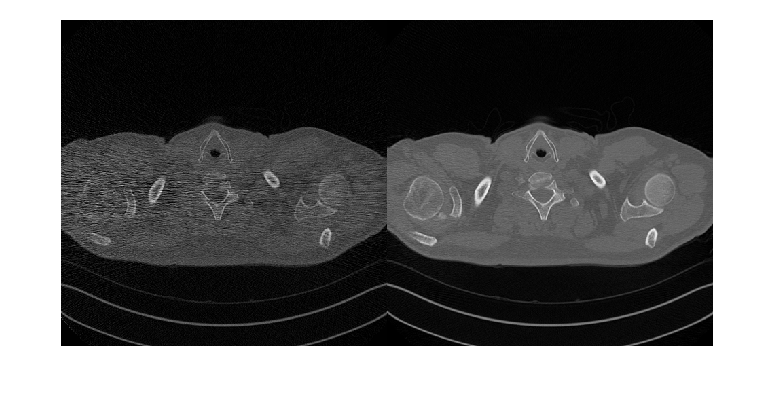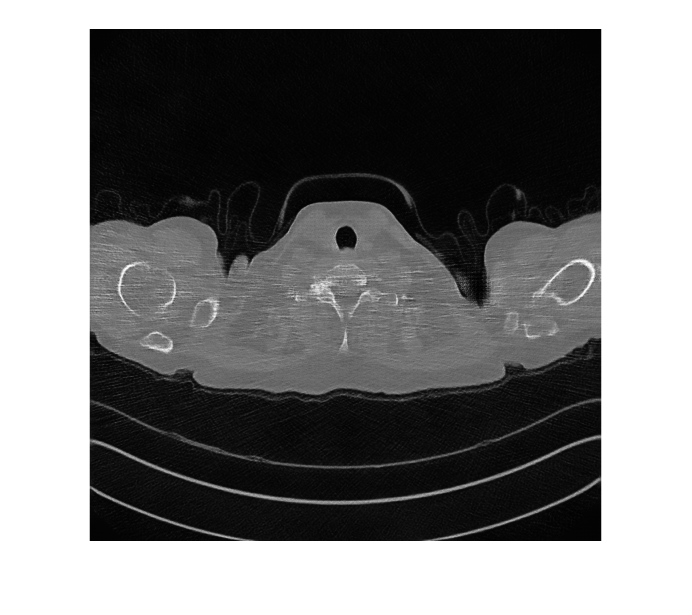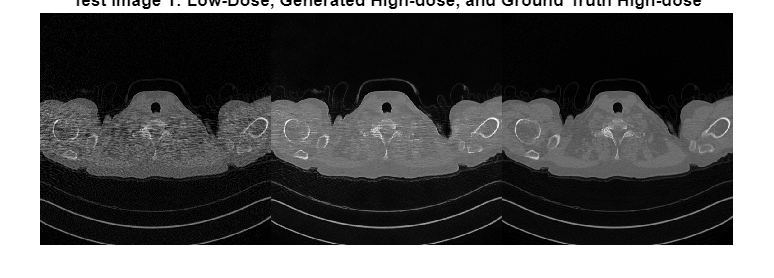# Unsupervised Medical Image Denoising Using UNIT

This example shows how to generate high-quality computed tomography (CT) images from noisy low-dose CT images using a UNIT neural network.

Note: This example references the Low Dose CT Grand Challenge data set, as accessed on May 1, 2021. The example uses chest images from the data set that are now under restricted access. To run this example, you must have a compatible data set with low-dose and high-dose CT images, and adapt the data preprocessing and training options to suit your data.

This example uses an unsupervised image-to-image translation (UNIT) neural network trained on full images from a limited sample of data. For a similar approach using a CycleGAN neural network trained on patches of image data from a large sample of data, see Unsupervised Medical Image Denoising Using CycleGAN (Image Processing Toolbox).

X-ray CT is a popular imaging modality used in clinical and industrial applications because it produces high-quality images and offers superior diagnostic capabilities. To protect the safety of patients, clinicians recommend a low radiation dose. However, a low radiation dose results in a lower signal-to-noise ratio (SNR) in the images, and therefore reduces the diagnostic accuracy.

Deep learning techniques offer solutions to improve the image quality for low-dose CT (LDCT) images. Using a generative adversarial network (GAN) for image-to-image translation, you can convert noisy LDCT images to images of the same quality as regular-dose CT images. For this application, the source domain consists of LDCT images and the target domain consists of regular-dose images.

CT image denoising requires a GAN that performs unsupervised training because clinicians do not typically acquire matching pairs of low-dose and regular-dose CT images of the same patient in the same session. This example uses a UNIT architecture that supports unsupervised training. For more information, see Get Started with GANs for Image-to-Image Translation (Image Processing Toolbox).

This example uses data from the Low Dose CT Grand Challenge [2, 3, 4]. The data includes pairs of regular-dose CT images and simulated low-dose CT images for 99 head scans (labeled N for neuro), 100 chest scans (labeled C for chest), and 100 abdomen scans (labeled L for liver).

Specify `dataDir` as the desired location of the data set. The data for this example requires 52 GB of memory.

`dataDir = fullfile(tempdir,"LDCT","LDCT-and-Projection-data");`

To download the data, go to the Cancer Imaging Archive website. This example uses only two patient scans from the chest. Download the chest files "C081" and "C120" from the "Images (DICOM, 952 GB)" data set using the NBIA Data Retriever. Specify the `dataFolder` variable as the location of the downloaded data. When the download is successful, `dataFolder` contains two subfolders named "C081" and "C120".

### Create Datastores for Training, Validation, and Testing

Specify the patient scans that are the source of each data set.

```scanDirTrain = fullfile(dataDir,"C120","08-30-2018-97899"); scanDirTest = fullfile(dataDir,"C081","08-29-2018-10762");```

Create `imageDatastore` objects that manage the low-dose and high-dose CT images for training and testing. The data set consists of DICOM images, so use the custom `ReadFcn` name-value argument in `imageDatastore` to enable reading the data.

```exts = ".dcm"; readFcn = @(x)rescale(dicomread(x)); imdsLDTrain = imageDatastore(fullfile(scanDirTrain,"1.000000-Low Dose Images-71581"), ... FileExtensions=exts,ReadFcn=readFcn); imdsHDTrain = imageDatastore(fullfile(scanDirTrain,"1.000000-Full dose images-34601"), ... FileExtensions=exts,ReadFcn=readFcn); imdsLDTest = imageDatastore(fullfile(scanDirTest,"1.000000-Low Dose Images-32837"), ... FileExtensions=exts,ReadFcn=readFcn); imdsHDTest = imageDatastore(fullfile(scanDirTest,"1.000000-Full dose images-95670"), ... FileExtensions=exts,ReadFcn=readFcn);```

Preview a training image from the low-dose and high-dose CT training data sets.

```lowDose = preview(imdsLDTrain); highDose = preview(imdsHDTrain); montage({lowDose,highDose})```### Preprocess and Augment Training Data

Specify the image input size for the source and target images.

`inputSize = [256,256,1];`

Augment and preprocess the training data by using the `transform` function with custom preprocessing operations specified by the `augmentDataForLD2HDCT` helper function. This function is attached to the example as a supporting file.

The `augmentDataForLD2HDCT` function performs these operations:

1. Resize the image to the specified input size using bicubic interpolation.

2. Randomly flip the image in the horizontal direction.

3. Scale the image to the range [-1, 1]. This range matches the range of the final `tanhLayer` used in the generator.

```imdsLDTrain = transform(imdsLDTrain, @(x)augmentDataForLD2HDCT(x,inputSize)); imdsHDTrain = transform(imdsHDTrain, @(x)augmentDataForLD2HDCT(x,inputSize));```

The LDCT data set provides pairs of low-dose and high-dose CT images. However, the UNIT architecture requires unpaired data for unsupervised learning. This example simulates unpaired training and validation data by shuffling the data in each iteration of the training loop.

#### Batch Training and Validation Data During Training

This example uses a custom training loop. The `minibatchqueue` object is useful for managing the mini-batching of observations in custom training loops. The `minibatchqueue` object also casts data to a `dlarray` object that enables auto differentiation in deep learning applications.

Specify the mini-batch data extraction format as `SSCB` (spatial, spatial, channel, batch). Set the `DispatchInBackground` name-value argument as the boolean returned by `canUseGPU`. If a supported GPU is available for computation, then the `minibatchqueue` object preprocesses mini-batches in the background in a parallel pool during training.

```miniBatchSize = 1; mbqLDTrain = minibatchqueue(imdsLDTrain,MiniBatchSize=miniBatchSize, ... MiniBatchFormat="SSCB",DispatchInBackground=canUseGPU); mbqHDTrain = minibatchqueue(imdsHDTrain,MiniBatchSize=miniBatchSize, ... MiniBatchFormat="SSCB",DispatchInBackground=canUseGPU);```

### Create Generator Network

The UNIT consists of one generator and two discriminators. The generator performs image-to-image translation from low dose to high dose. The discriminators are PatchGAN networks that return the patch-wise probability that the input data is real or generated. One discriminator distinguishes between the real and generated low-dose images and the other discriminator distinguishes between real and generated high-dose images.

Create a UNIT generator network using the `unitGenerator` (Image Processing Toolbox) function. The source and target encoder sections of the generator each consist of two downsampling blocks and five residual blocks. The encoder sections share two of the five residual blocks. Likewise, the source and target decoder sections of the generator each consist of two downsampling blocks and five residual blocks, and the decoder sections share two of the five residual blocks.

`gen = unitGenerator(inputSize);`

Visualize the generator network.

`analyzeNetwork(gen)`

### Create Discriminator Networks

There are two discriminator networks, one for each of the image domains (low-dose CT and high-dose CT). Create the discriminators for the source and target domains using the `patchGANDiscriminator` (Image Processing Toolbox) function.

```discLD = patchGANDiscriminator(inputSize,NumDownsamplingBlocks=4,FilterSize=3, ... ConvolutionWeightsInitializer="narrow-normal",NormalizationLayer="none"); discHD = patchGANDiscriminator(inputSize,"NumDownsamplingBlocks",4,FilterSize=3, ... ConvolutionWeightsInitializer="narrow-normal",NormalizationLayer="none");```

Visualize the discriminator networks.

```analyzeNetwork(discLD); analyzeNetwork(discHD);```

### Define Model Gradients and Loss Functions

The `modelGradientDisc` and `modelGradientGen` helper functions calculate the gradients and losses for the discriminators and generator, respectively. These functions are defined in the Supporting Functions section of this example.

The objective of each discriminator is to correctly distinguish between real images (1) and translated images (0) for images in its domain. Each discriminator has a single loss function.

The objective of the generator is to generate translated images that the discriminators classify as real. The generator loss is a weighted sum of five types of losses: self-reconstruction loss, cycle consistency loss, hidden KL loss, cycle hidden KL loss, and adversarial loss.

Specify the weight factors for the various losses.

```lossWeights.selfReconLossWeight = 10; lossWeights.hiddenKLLossWeight = 0.01; lossWeights.cycleConsisLossWeight = 10; lossWeights.cycleHiddenKLLossWeight = 0.01; lossWeights.advLossWeight = 1; lossWeights.discLossWeight = 0.5;```

### Specify Training Options

Specify the options for Adam optimization. Train the network for 26 epochs.

`numEpochs = 26;`

Specify identical options for the generator and discriminator networks.

• Specify a learning rate of 0.0001.

• Initialize the trailing average gradient and trailing average gradient-square decay rates with `[]`.

• Use a gradient decay factor of 0.5 and a squared gradient decay factor of 0.999.

• Use weight decay regularization with a factor of 0.0001.

• Use a mini-batch size of 1 for training.

```learnRate = 0.0001; gradDecay = 0.5; sqGradDecay = 0.999; weightDecay = 0.0001; genAvgGradient = []; genAvgGradientSq = []; discLDAvgGradient = []; discLDAvgGradientSq = []; discHDAvgGradient = []; discHDAvgGradientSq = [];```

By default, the example downloads a pretrained version of the UNIT generator for the NIH-AAPM-Mayo Clinic Low-Dose CT data set. The pretrained network enables you to run the entire example without waiting for training to complete.

To train the network, set the `doTraining` variable in the following code to `true`. Train the model in a custom training loop. For each iteration:

• Read the data for the current mini-batch using the `next` function.

• Evaluate the discriminator model gradients using the `dlfeval` function and the `modelGradientDisc` helper function.

• Update the parameters of the discriminator networks using the `adamupdate` function.

• Evaluate the generator model gradients using the `dlfeval` function and the `modelGradientGen` helper function.

• Update the parameters of the generator network using the `adamupdate` function.

• Display the input and translated images for both the source and target domains after each epoch.

Train on a GPU if one is available. Using a GPU requires Parallel Computing Toolbox™ and a CUDA® enabled NVIDIA® GPU. For more information, see GPU Computing Requirements (Parallel Computing Toolbox). Training takes about 58 hours on an NVIDIA Titan RTX.

```doTraining = false; if doTraining % Create a figure to show the results figure(Units="Normalized"); for iPlot = 1:4 ax(iPlot) = subplot(2,2,iPlot); end iteration = 0; % Loop over epochs for epoch = 1:numEpochs % Shuffle data every epoch reset(mbqLDTrain); shuffle(mbqLDTrain); reset(mbqHDTrain); shuffle(mbqHDTrain); % Run the loop until all the images in the mini-batch queue % mbqLDTrain are processed while hasdata(mbqLDTrain) iteration = iteration + 1; % Read data from the low-dose domain imLowDose = next(mbqLDTrain); % Read data from the high-dose domain if hasdata(mbqHDTrain) == 0 reset(mbqHDTrain); shuffle(mbqHDTrain); end imHighDose = next(mbqHDTrain); % Calculate discriminator gradients and losses [discLDGrads,discHDGrads,discLDLoss,discHDLoss] = dlfeval(@modelGradientDisc, ... gen,discLD,discHD,imLowDose,imHighDose,lossWeights.discLossWeight); % Apply weight decay regularization on low-dose discriminator gradients discLDGrads = dlupdate(@(g,w) g+weightDecay*w,discLDGrads,discLD.Learnables); % Update parameters of low-dose discriminator [discLD,discLDAvgGradient,discLDAvgGradientSq] = adamupdate(discLD,discLDGrads, ... discLDAvgGradient,discLDAvgGradientSq,iteration,learnRate,gradDecay,sqGradDecay); % Apply weight decay regularization on high-dose discriminator gradients discHDGrads = dlupdate(@(g,w) g+weightDecay*w,discHDGrads,discHD.Learnables); % Update parameters of high-dose discriminator [discHD,discHDAvgGradient,discHDAvgGradientSq] = adamupdate(discHD,discHDGrads, ... discHDAvgGradient,discHDAvgGradientSq,iteration,learnRate,gradDecay,sqGradDecay); % Calculate generator gradient and loss [genGrad,genLoss,images] = dlfeval(@modelGradientGen,gen,discLD,discHD,imLowDose,imHighDose,lossWeights); % Apply weight decay regularization on generator gradients genGrad = dlupdate(@(g,w) g+weightDecay*w,genGrad,gen.Learnables); % Update parameters of generator [gen,genAvgGradient,genAvgGradientSq] = adamupdate(gen,genGrad,genAvgGradient, ... genAvgGradientSq,iteration,learnRate,gradDecay,sqGradDecay); end % Display the results updateTrainingPlotLD2HDCT_UNIT(ax,images{:}); end % Save the trained network modelDateTime = string(datetime("now",Format="yyyy-MM-dd-HH-mm-ss")); save(fullfile(dataDir,"trainedLowDoseHighDoseUNITGeneratorNet-"+modelDateTime+".mat"),"gen"); else net_url = "https://ssd.mathworks.com/supportfiles/vision/data/trainedLowDoseHighDoseUNITGeneratorNet.zip"; downloadTrainedNetwork(net_url,dataDir); load(fullfile(dataDir,"trainedLowDoseHighDoseUNITGeneratorNet.mat")); end```

### Generate High-Dose Image Using Trained Network

Read and display an image from the datastore of low-dose test images.

```idxToTest = 1; imLowDoseTest = readimage(imdsLDTest,idxToTest); figure imshow(imLowDoseTest)```Convert the image to data type `single`. Rescale the image data to the range [-1, 1] as expected by the final layer of the generator network.

```imLowDoseTest = im2single(imLowDoseTest); imLowDoseTestRescaled = (imLowDoseTest-0.5)/0.5;```

Create a `dlarray` object that inputs data to the generator. If a supported GPU is available for computation, then perform inference on a GPU by converting the data to a `gpuArray` object.

```dlLowDoseImage = dlarray(imLowDoseTestRescaled,'SSCB'); if canUseGPU dlLowDoseImage = gpuArray(dlLowDoseImage); end```

Translate the input low-dose image to the high-dose domain using the `unitPredict` (Image Processing Toolbox) function. The generated image has pixel values in the range [-1, 1]. For display, rescale the activations to the range [0, 1].

```dlImLowDoseToHighDose = unitPredict(gen,dlLowDoseImage); imHighDoseGenerated = extractdata(gather(dlImLowDoseToHighDose)); imHighDoseGenerated = rescale(imHighDoseGenerated); imshow(imHighDoseGenerated)```Read and display the ground truth high-dose image. The high-dose and low-dose test datastores are not shuffled, so the ground truth high-dose image corresponds directly to the low-dose test image.

```imHighDoseGroundTruth = readimage(imdsHDTest,idxToTest); imshow(imHighDoseGroundTruth)```Display the input low-dose CT, the generated high-dose version, and the ground truth high-dose image in a montage. Although the network is trained on data from a single patient scan, the network generalizes well to test images from other patient scans.

```imshow([imLowDoseTest imHighDoseGenerated imHighDoseGroundTruth]) title("Test Image "+num2str(idxToTest)+": Low-Dose, Generated High-dose, and Ground Truth High-dose")```### Supporting Functions

The `modelGradientGen` helper function calculates the gradients and loss for the generator.

```function [genGrad,genLoss,images] = modelGradientGen(gen,discLD,discHD,imLD,imHD,lossWeights) [imLD2LD,imHD2LD,imLD2HD,imHD2HD] = forward(gen,imLD,imHD); hidden = forward(gen,imLD,imHD,Outputs="encoderSharedBlock"); [~,imLD2HD2LD,imHD2LD2HD,~] = forward(gen,imHD2LD,imLD2HD); cycle_hidden = forward(gen,imHD2LD,imLD2HD,Outputs="encoderSharedBlock"); % Calculate different losses selfReconLoss = computeReconLoss(imLD,imLD2LD) + computeReconLoss(imHD,imHD2HD); hiddenKLLoss = computeKLLoss(hidden); cycleReconLoss = computeReconLoss(imLD,imLD2HD2LD) + computeReconLoss(imHD,imHD2LD2HD); cycleHiddenKLLoss = computeKLLoss(cycle_hidden); outA = forward(discLD,imHD2LD); outB = forward(discHD,imLD2HD); advLoss = computeAdvLoss(outA) + computeAdvLoss(outB); % Calculate the total loss of generator as a weighted sum of five losses genTotalLoss = ... selfReconLoss*lossWeights.selfReconLossWeight + ... hiddenKLLoss*lossWeights.hiddenKLLossWeight + ... cycleReconLoss*lossWeights.cycleConsisLossWeight + ... cycleHiddenKLLoss*lossWeights.cycleHiddenKLLossWeight + ... advLoss*lossWeights.advLossWeight; % Update the parameters of generator genGrad = dlgradient(genTotalLoss,gen.Learnables); % Convert the data type from dlarray to single genLoss = extractdata(genTotalLoss); images = {imLD,imLD2HD,imHD,imHD2LD}; end```

The `modelGradientDisc` helper function calculates the gradients and loss for the two discriminators.

```function [discLDGrads,discHDGrads,discLDLoss,discHDLoss] = modelGradientDisc(gen, ... discLD,discHD,imRealLD,imRealHD,discLossWeight) [~,imFakeLD,imFakeHD,~] = forward(gen,imRealLD,imRealHD); % Calculate loss of the discriminator for low-dose images outRealLD = forward(discLD,imRealLD); outFakeLD = forward(discLD,imFakeLD); discLDLoss = discLossWeight*computeDiscLoss(outRealLD,outFakeLD); % Update parameters of the discriminator for low-dose images discLDGrads = dlgradient(discLDLoss,discLD.Learnables); % Calculate loss of the discriminator for high-dose images outRealHD = forward(discHD,imRealHD); outFakeHD = forward(discHD,imFakeHD); discHDLoss = discLossWeight*computeDiscLoss(outRealHD,outFakeHD); % Update parameters of the discriminator for high-dose images discHDGrads = dlgradient(discHDLoss,discHD.Learnables); % Convert the data type from dlarray to single discLDLoss = extractdata(discLDLoss); discHDLoss = extractdata(discHDLoss); end```

#### Loss Functions

The `computeDiscLoss` helper function calculates discriminator loss. Each discriminator loss is a sum of two components:

• The squared difference between a vector of ones and the predictions of the discriminator on real images, ${\mathit{Y}}_{\mathit{real}}$

• The squared difference between a vector of zeros and the predictions of the discriminator on generated images, ${\stackrel{ˆ}{\mathit{Y}}}_{\mathit{translated}}$

`$\mathit{discriminatorLoss}={\left(1-{\mathit{Y}}_{\mathit{real}}\right)}^{2}+{\left(0-{\stackrel{ˆ}{\mathit{Y}}}_{\mathit{translated}}\right)}^{2}$`

```function discLoss = computeDiscLoss(Yreal,Ytranslated) discLoss = mean(((1-Yreal).^2),"all") + ... mean(((0-Ytranslated).^2),"all"); end```

The `computeAdvLoss` helper function calculates adversarial loss for the generator. Adversarial loss is the squared difference between a vector of ones and the discriminator predictions on the translated image.

`$\mathit{adversarialLoss}={\left(1-{\stackrel{ˆ}{\mathit{Y}}}_{\mathit{translated}}\right)}^{2}$`

```function advLoss = computeAdvLoss(Ytranslated) advLoss = mean(((Ytranslated-1).^2),"all"); end```

The `computeReconLoss` helper function calculates self-reconstruction loss and cycle consistency loss for the generator. Self reconstruction loss is the ${\mathit{L}}^{1}$ distance between the input images and their self-reconstructed versions. Cycle consistency loss is the ${\mathit{L}}^{1}$ distance between the input images and their cycle-reconstructed versions.

`$\mathit{selfReconstructionLoss}={‖\left({\mathit{Y}}_{\mathit{real}}-{\mathit{Y}}_{\mathit{self}-\mathit{reconstructed}}\right)‖}_{1}$`

`$\mathit{cycleConsistencyLoss}={‖\left({\mathit{Y}}_{\mathit{real}}-{\mathit{Y}}_{\mathit{cycle}-\mathit{reconstructed}}\right)‖}_{1}$`

```function reconLoss = computeReconLoss(Yreal,Yrecon) reconLoss = mean(abs(Yreal-Yrecon),"all"); end```

The `computeKLLoss` helper function calculates hidden KL loss and cycle-hidden KL loss for the generator. Hidden KL loss is the squared difference between a vector of zeros and the '`encoderSharedBlock`' activation for the self-reconstruction stream. Cycle-hidden KL loss is the squared difference between a vector of zeros and the '`encoderSharedBlock`' activation for the cycle-reconstruction stream.

`$\mathit{hiddenKLLoss}={\left(0-{\mathit{Y}}_{\mathit{encoderSharedBlockActivation}}\right)}^{2}$`

`$\mathit{cycleHiddenKLLoss}={\left(0-{\mathit{Y}}_{\mathit{encoderSharedBlockActivation}}\right)}^{2}$`

```function klLoss = computeKLLoss(hidden) klLoss = mean(abs(hidden.^2),"all"); end```

### References

 Liu, Ming-Yu, Thomas Breuel, and Jan Kautz, "Unsupervised image-to-image translation networks". In Advances in Neural Information Processing Systems, 2017. https://arxiv.org/pdf/1703.00848.pdf.

 McCollough, C.H., Chen, B., Holmes, D., III, Duan, X., Yu, Z., Yu, L., Leng, S., Fletcher, J. (2020). Data from Low Dose CT Image and Projection Data [Data set]. The Cancer Imaging Archive. https://doi.org/10.7937/9npb-2637.

 Grants EB017095 and EB017185 (Cynthia McCollough, PI) from the National Institute of Biomedical Imaging and Bioengineering.

 Clark, Kenneth, Bruce Vendt, Kirk Smith, John Freymann, Justin Kirby, Paul Koppel, Stephen Moore, et al. “The Cancer Imaging Archive (TCIA): Maintaining and Operating a Public Information Repository.” Journal of Digital Imaging 26, no. 6 (December 2013): 1045–57. https://doi.org/10.1007/s10278-013-9622-7.